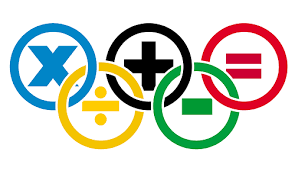Một số phép toán cơ bản trong toán họceg: 1+2=3

a.one and two is three.

b.one and two are three.

c.one and two makes three

d. one plus two equals three.(*)

2. subtraction: phép trừ

eg: 2-1=1

one from two is one.

two minus one equals one.(*)

3. multiplication: phép nhân

eg: 5x6=30

a. five sixes are thirtychúng ta thêm đuôi es vào thừa số thứ 2

b. five times six is/equals thirty.

c. five multiplied six equals thirty(*)

4. division

20:4=5

a. four into twenty goes five.

b. twenty divided by four is/equals five(*)

5. point: số thập phân

eg: 360:50=7.2

Three hundred and sixty divided by fifty equals seven point two

Lan Anh

ĐĂNG KÝ HỌC TIẾNG ANH TẠI EPASS (TP.HCM) theo link này: http://goo.gl/forms/IzBHuhdMUn

MỌI THẮC MẮC VỀ VIỆC HỌC TIẾNG ANH & DỊCH VỤ VISA ĐI MỸ - ÚC - CANADA. LIÊN HỆ NGAY:

Địa chỉ: 421/37 Sư Vạn Hạnh (nối dài), Phường 12, Quận 10, TP.HCM

ĐT: 08.6270.8.666 - Hotline: 0915 332 966 (Thầy Tấn) - 0982 223 844 (Cô Mỹ Hà)

Email: epass@outlook.com.vn

Website: www.epass.com.vn